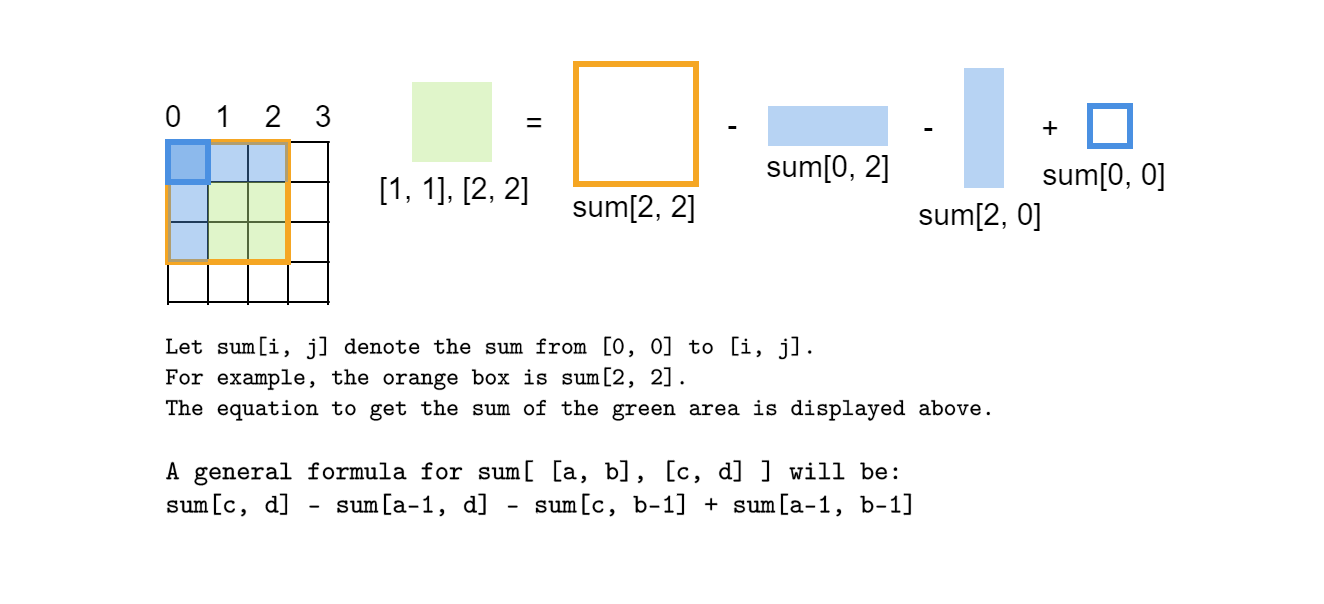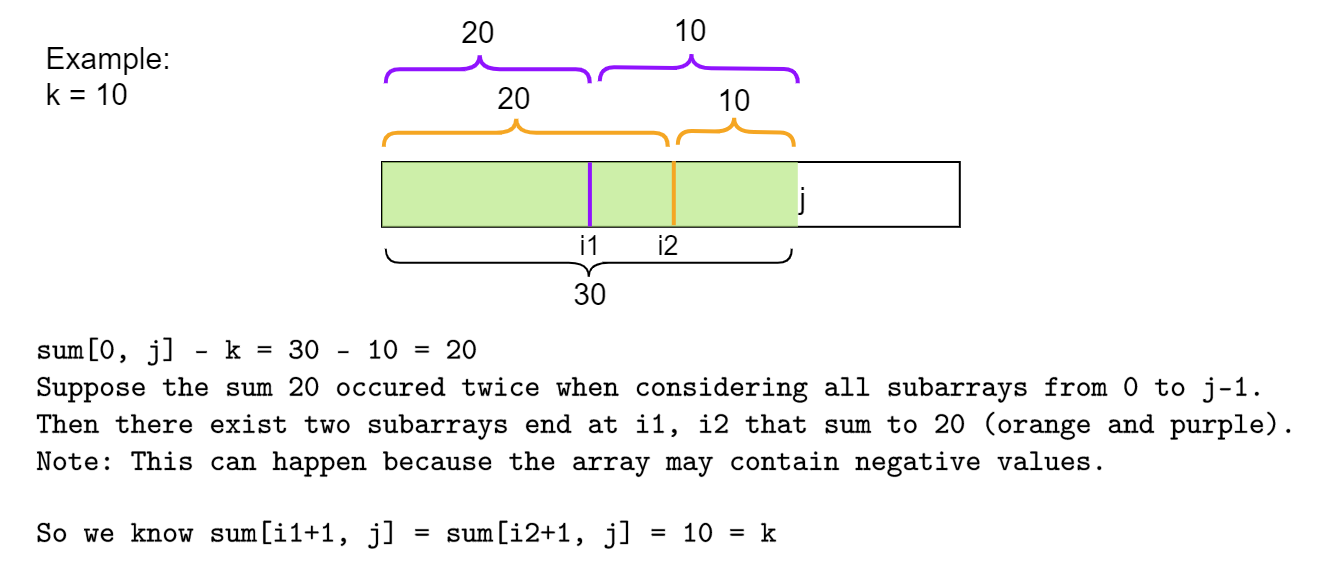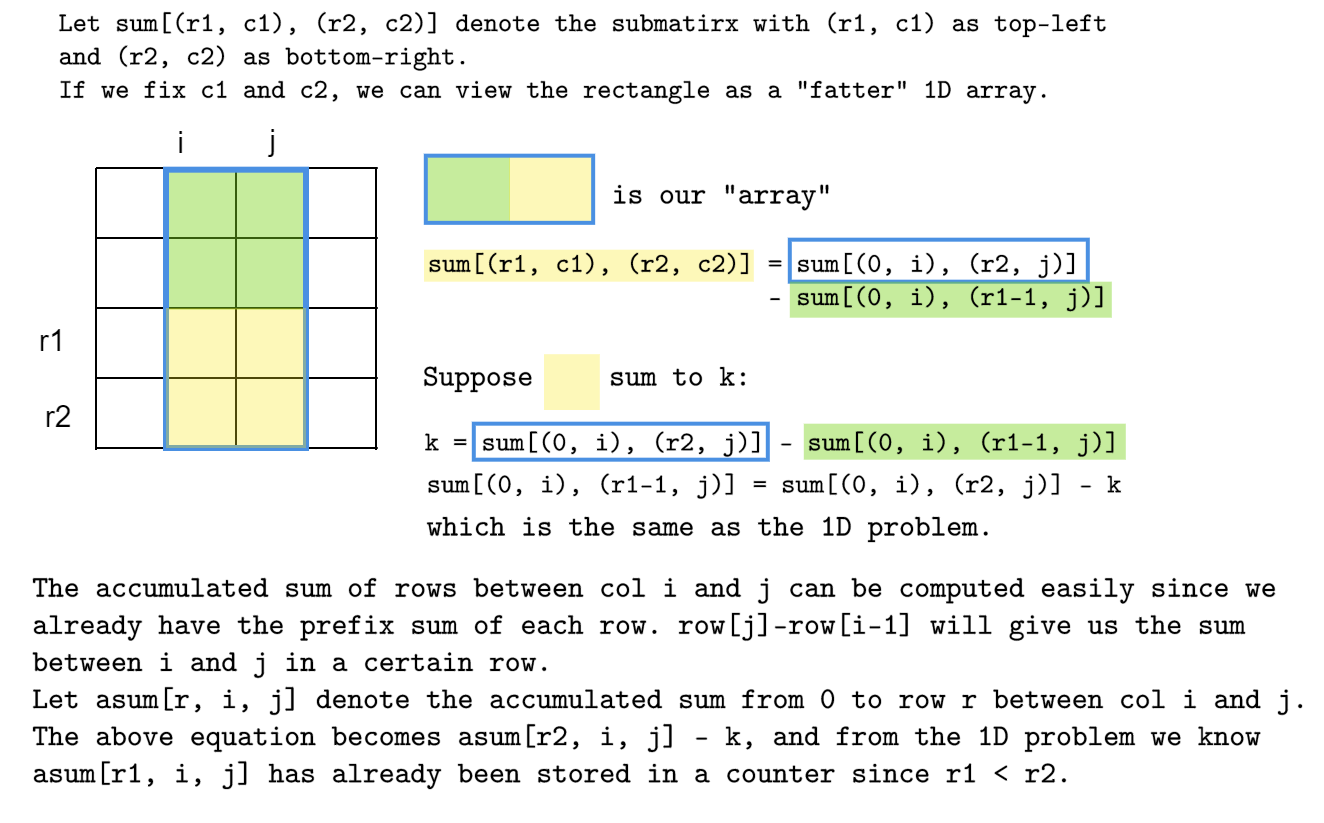# Sixian Li

## Number of submatrices that sum to target

Algorithm, LeetCode

## Brute force

Consider every possible [a, b](top-left position) and [c, d] (bottom-right position) pair, and check their sums.

### Time complexity

Without loss of generality, we assume the given matrix is a square matrix with size nxn.

For each a, we have n choices for b, and we have n choices for a —> $n^2$.

For each [a, b], we have n*n pairs of [c, d]. —> $n^2 * n^2 = n^4$

However, each time we need to sum up all entries in the submatrix, giving us $O(n^6)$. This is unacceptable for large inputs.

## Optimize the brute force strategy

Do we need to sum up all entries in a submatrix every time? Can we reuse some computations?

Observation from a simple case: if the “height” of the matrix is 1, it is essentially an array. Let sum[i] denote the sum of the subarray from index 0 to i. If we want to know sum, we only need to add the third element to sum. So we can store sum[i], and the addition only costs $O(1)$.

We can extend the idea to 2D to reuse computations:To get sum[i, j], we use the following loop:

### Time complexity

Now, we can get the sum of any submatrix in $O(1)$ use the formula, but we still have $O(n^4)$ pairs of “top-left” and “bottom-right” which can’t be reduced further. The total cost is $O(n^4)$.

## “Compress” rows to 1D

### Number of subarrays that sum to target

It’s always easier to start with a simpler version of the problem and extend the idea.

The 1D counterpart of this problem is “number of subarrays that sum to target”.

The idea is from the discussion of 560. Subarray Sum Equals K . It is amazing how a key observation reduced the cost to $O(n)$.

Let sum[i, j] denote the sum from index i to j.

We observe that the any sum[i, j] can be broken into two parts:

\begin{aligned} \text{sum}[i, j] &= \text{sum}[0, j] - \text{sum}[i-1]\\ \text{Assume sum}[i, j]& = k, \text{the given target}\\ k &= \text{sum}[0, j] - \text{sum}[i-1]\\ \text{sum}[i-1] &=\text{sum}[0, j]-k \end{aligned}The key observation is: #occurrences of sum[0, j]-k is equivalent to the number of subarrays that sum to k among all possible subarrays between 0 and j. Let’s consider an example:### From 1D to 2D

If we can control one dimension, we will reduce the matrix problem to the simpler array problem.

We first compute the prefix sum for each row, and we fix col i an col j to “convert” the submatrix to a subarray.### Time complexity

1. Get prefix sum for each row —> $O(n^2)$
2. For each pair of (i, j), we need $O(n)$ to determine the submatrices. There are $O(n^2)$ pairs —> $O(n^3)$

Total cost: $O(n^3)$

The similar idea of manipulating the equation can also be applied to any problem associated with “find the number of sub-patterns that satisfy a given condition”.